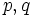# Proving join-closedness

This is a survey article related to:subgroup metaproperty satisfaction
View other survey articles about subgroup metaproperty satisfaction

A subgroup property$p$ is termed a join-closed subgroup property if the join of subgroups of a group, each of which satisfies property$p$ in the group, also satisfies$p$ in the group.$p$ is a strongly join-closed subgroup property if it is both a join-closed subgroup property and a trivially true subgroup property: it is satisfied by the trivial subgroup in any group.$p$ is a finite-join-closed subgroup property if it is closed under joins of finitely many subgroups. It is a strongly finite-join-closed subgroup property if it is both finite-join-closed and trivially true.

This article discusses methods to prove that a subgroup property is join-closed.

Also refer:

## Endo-invariance properties

Further information: Endo-invariance implies strongly join-closed

Suppose$a$ is a property of functions from a group to itself. The invariance property corresponding to$a$ is the subgroup property$p$ defined as follows:$H$ satisfies$p$ in$G$ if, for any function$f$ from$G$ to itself satisfying property$a$,$f(H) \subseteq H$.

An endo-invariance property is an invariance property corresponding to a property of functions that is only true for endomorphisms.

It turns out that any endo-invariance property is strongly join-closed: it is satisfied by the trivial subgroup and an arbitrary join of subgroups satisfying the property also satisfies the property.

Here are some examples:

## Upward-closed subgroup properties

A subgroup property is termed an upward-closed subgroup property if, whenever$H$ is a subgroup of$G$ satisfying the property, any subgroup of$G$ containing$H$ also satisfies the property. Here are some examples:

## Effect of logical operators

### Conjunction

The conjunction (AND) of finite-join-closed subgroup properties is again finite-join-closed. Similarly, the conjunction of join-closed subgroup properties is also join-closed.

### Disjunction

The disjunction (OR) of join-closed subgroup properties need not be join-closed. Similarly, the disjunction of finite-join-closed subgroup properties need not be finite-join-closed.

## Effect of subgroup property modifiers

### Join-transiter

Suppose$p$ is a subgroup property. The join-transiter of$p$ is the property of being a subgroup whose join with any subgroup having property$p$ still has property$p$.

The join-transiter of any subgroup property is a strongly finite-join-closed subgroup property.

Some examples are:

### Join-closure

The finite-join-closure operator takes as input a subgroup property and outputs the property of being a join of finitely many subgroups satisfying that property. The finite-join-closure operator applied to any property gives a finite-join-closed subgroup property.

The join-closure operator takes as input a subgroup property and outputs the property of being a join of (possibly infinitely) many subgroups satisfying the property. The join-closure operator applied to any property gives a join-closed subgroup property.

### Join operator

The join operator is a binary operator that takes as input two subgroup properties. Suppose$p,q$ are subgroup properties. A subgroup$H$ of a group$G$ is said to satisfy the property obtained by the join operator on$p$ and$q$ if there exist subgroups$K,L \le G$ such that$H = \langle K, L \rangle$.

The following are true:

• The join operator applied to two finite-join-closed subgroup properties is also finite-join-closed.
• The join operator applied to two join-closed subgroup properties is also join-closed.